# OceanBase 4.0 解读：分布式查询性能提升，我们是如何思考的？

• 2022-11-25
浙江
• 本文字数：12433 字

阅读完需：约 41 分钟OceanBase 高级技术专家

## OceanBase 4.0 如何做分布式查询优化 ？

### ▋ OceanBase 3.x 的二阶段分布式查询优化方法

create table R1(a int primary key, b int, c int, d int) partition by hash(a) partitions 4;create table R2(a int primary key, b int, c int, d int) partition by hash(a) partitions 4;create table R3(a int primary key, b int, c int, d int) partition by hash(b) partitions 5;select R2.c, sum(R3.d) from R1, R2, R3 where R1.a = R2.a and R2.C = R3.C group by R2.C;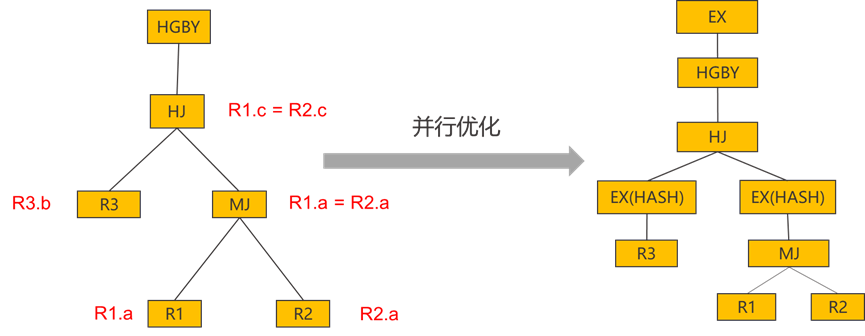### 没有考虑分区信息导致选择了不优的本地算法

create table R1(a int primary key, b int, c int);create table R2(a int primary key, b int, c int, index idx(b)) partition by hash(a) partitions 100;Q2: select * from R1, R2 where R2.b = R1.b and R1.c = 100;/*一阶段计划*/| =============================================|ID|OPERATOR        |NAME   |EST. ROWS|COST |---------------------------------------------|0 |NESTED-LOOP JOIN|       |970299   |85622||1 | TABLE SCAN     |r1     |990      |40790||2 | TABLE SCAN     |r2(idx)|1        |44   |=============================================Outputs & filters:-------------------------------------  0 - output([r1.a], [r1.b], [r1.c], [r2.a], [r2.b], [r2.c]), filter(nil),      conds(nil), nl_params_([r1.b])  1 - output([r1.b], [r1.c], [r1.a]), filter([r1.c = 100]),      access([r1.b], [r1.c], [r1.a]), partitions(p0)  2 - output([r2.b], [r2.a], [r2.c]), filter(nil),      access([r2.b], [r2.a], [r2.c]), partitions(p0)

#### 没有考虑分区信息导致选择了不优的连接顺序

create table R1(a int primary key, b int, c int, d int) partition by hash(a) partitions 4;create table R2(a int primary key, b int, c int, d int) partition by hash(a) partitions 4;create table R3(a int primary key, b int, c int, d int) partition by hash(b) partitions 5;Q3: select R2.c, sum(R3.d) from R1, R2, R3 where R1.a = R2.a and R2.b = R3.b;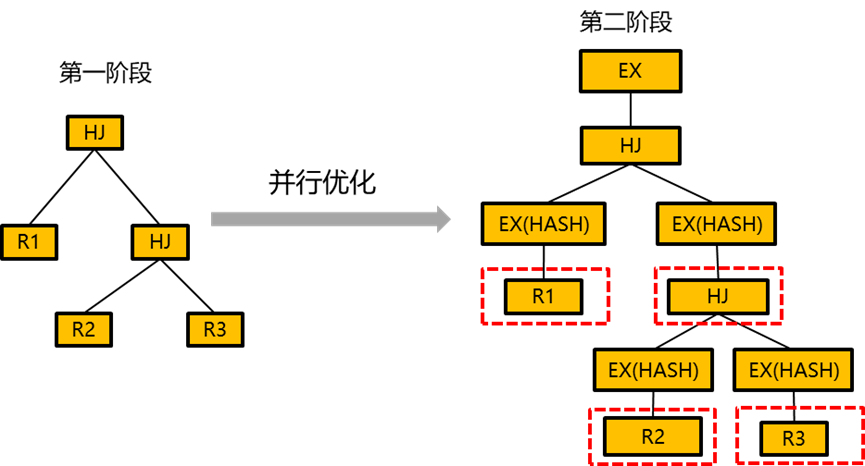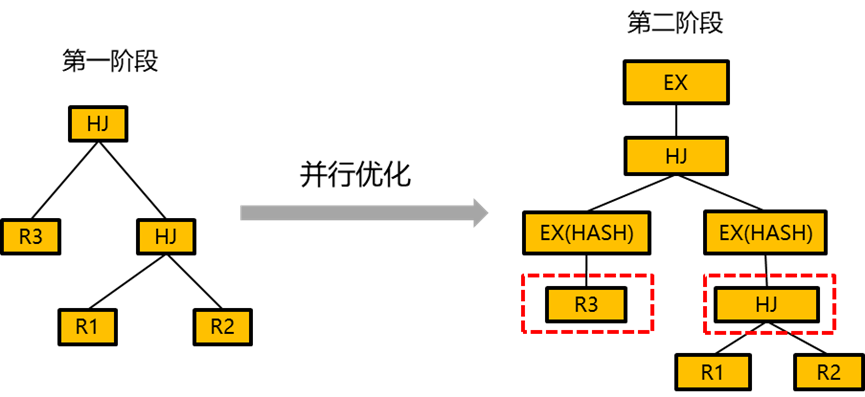### ▋ OceanBase 4.0 的分布式查询优化

• 枚举所有单机的连接算法，维护序这个物理属性，使用单机代价模型来计算代价。

• 保留代价最小的计划和存在有用序的计划，一个计划的序是有用的当且仅当该序对后续算子的分配有用。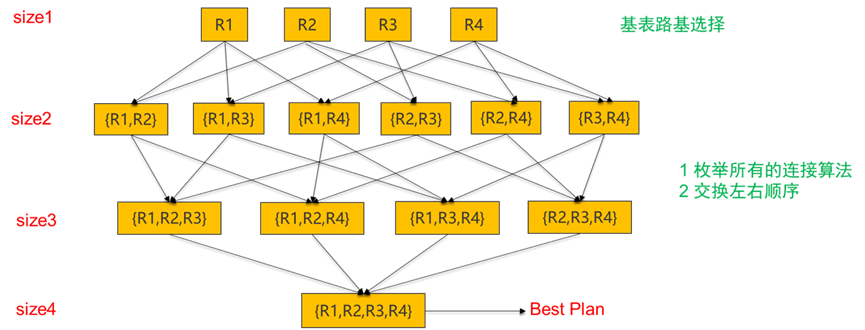1. 对于每一个枚举的子集，枚举所有算子的分布式算法，对于每一个分布式算法，OceanBase 使用分布式代价模型来计算代价，同时 OceanBase 会同时维护序和分区信息这两个物理属性。

2. 对于每一个枚举子集，除了保留代价最小的计划，保留存在有用序的计划，同时还需要保留有存在有用分区信息的计划。一个分区信息是有用的当且仅当它对后续的算子有用。考虑下图所示的场景, 在该场景中，P1 采用了 HASH-HASH 重分区的 HASH JOIN 方法, P2 采用了对 R2 做 BROADCAST 的 HASH JOIN 方法，虽然 P2 的代价比 P1 的代价高，但是 P2 继承了 R1 的分区信息，对后续的 group by 算子是有用的，因此 P2 这个计划也会被保留。

create table R1(a int primary key, b int, c int, d int) partition by hash(a) partitions 4;create table R2(a int primary key, b int, c int, d int) partition by hash(a) partitions 4;select R1.a, SUM(R2.c) from R1, R2 where R1.b = R2.b group by R1.a;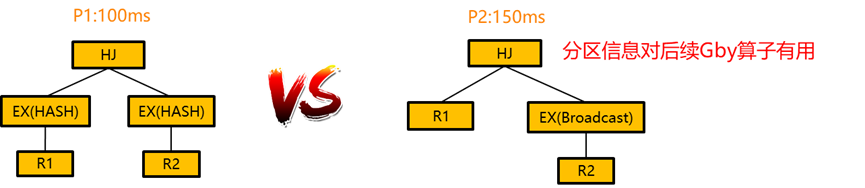OceanBase 4.0 使用了一阶段的分布式查询优化方法，相比于单机的查询优化，分布式查询优化的计划空间是非常大的。为了解决计划空间大的问题，OceanBase 4.0 发明了很多快速裁剪计划的方法以及新增了新的连接枚举算法来支持超大规模表的分布式计划枚举。 通过这些技术，OceanBase 4.0 大大减少了分布式计划空间，提升了分布式查询优化的性能。同时我们的实验结果也表明 OceanBase 4.0 可以在秒级内完成 50 张表的分布式计划的枚举。

## OceanBase 4.0 如何提升分布式执行引擎性能 ？

### ▋ OceanBase 4.0 执行引擎开始朝着自适应的方向发展

OceanBase 4.0 实现了自适应的 Group by/Distinct 并行下压技术，它可以解决 Group by/Distinct 并行下压场景中因为计划不优而导致的性能回退问题。在正式介绍该自适应技术之前，我们首先简单介绍一下 Group by/Distinct 并行下压技术。Group by/Distinct 并行下压技术是分布式执行中一种常见的并行下压技术，它的核心思想是提前把 Group by 算子下压下去做部分的数据预聚合，通过预聚合的方式可以减少网络传输从而达到提升性能的目的。 下图展示了一个 Group by 并行下压的执行计划的例子，其中 5 号算子就是下压的 Group by 算子，通过 5 号算子的预聚合可以减少 4 号算子网络传输从而达到性能提升的目的。但是这里需要注意的是 Group by 并行下压不一定会带来性能上的提升，有时候也会导致性能回退，主要原因是因为下压的 Group By 算子会引来额外的计算代价，所以只有当网络传输带来的性能提升超过下压的 Group By 带来的计算开销，Group by 的并行下压才会带来收益。

create table R1(a int primary key, b int, c int) partition by hash(a) partitions 4;explain select b, sum(c) from R1 group by b;| ==========================================================|ID|OPERATOR                     |NAME    |EST. ROWS|COST|----------------------------------------------------------|0 |PX COORDINATOR               |        |1        |10  ||1 | EXCHANGE OUT DISTR          |:EX10001|1        |10  ||2 |  HASH GROUP BY              |        |1        |9   ||3 |   EXCHANGE IN DISTR         |        |1        |9   ||4 |    EXCHANGE OUT DISTR (HASH)|:EX10000|1        |8   ||5 |     HASH GROUP BY           |        |1        |8   ||6 |      PX PARTITION ITERATOR  |        |1        |7   ||7 |       TABLE SCAN            |r1      |1        |7   |==========================================================Outputs & filters:-------------------------------------  0 - output([INTERNAL_FUNCTION(r1.b, T_FUN_SUM(T_FUN_SUM(r1.c)))]), filter(nil), rowset=256  1 - output([INTERNAL_FUNCTION(r1.b, T_FUN_SUM(T_FUN_SUM(r1.c)))]), filter(nil), rowset=256, dop=1  2 - output([r1.b], [T_FUN_SUM(T_FUN_SUM(r1.c))]), filter(nil), rowset=256,      group([r1.b]), agg_func([T_FUN_SUM(T_FUN_SUM(r1.c))])  3 - output([r1.b], [T_FUN_SUM(r1.c)]), filter(nil), rowset=256  4 - (#keys=1, [r1.b]), output([r1.b], [T_FUN_SUM(r1.c)]), filter(nil), rowset=256, dop=1  5 - output([r1.b], [T_FUN_SUM(r1.c)]), filter(nil), rowset=256,      group([r1.b]), agg_func([T_FUN_SUM(r1.c)])  6 - output([r1.b], [r1.c]), filter(nil), rowset=256  7 - output([r1.b], [r1.c]), filter(nil), rowset=256,      access([r1.b], [r1.c]), partitions(p[0-3])

OceanBase 在之前的版本中都是优化器通过计算代价来决定是否要下压 Group by 算子，但是因为优化器有时会错误的估计行数，会导致出现没有正确的下压 Group by 算子或者错误的下压了 Group by 算子的场景，最终导致执行性能次优。为了解决这个问题，OceanBase 4.0 引入了自适应的 Group by/Distinct 并行下压技术，其核心思想是让优化器总是下压 Group by/Distinct 算子，然后在执行的时候通过采样下压算子的一部分数据来决定是否跳过下压的 Group by/Distinct 算子。该技术的难点在于如何判断下压的算子是否具备足够好的预聚合能力。OceanBase 采用了控制下压算子的 HASH 表在 L3 cache 之内(控制 Hash 表的性能)以及多轮采样的策略(确保数据连续非聚合性带来的误判)来判断下压算子是否具备足够好的预聚合能力。其核心思想如下:

• 下压算子 hash 表尽量维持在 L2 cache (1M) 内, 如果预聚合效果不好，标记该 hash 表状态为舍弃。如果预聚合效果很好, 可以将 hash 表扩张到 L3 cache(10 M)，如果执行过程中发现需要更大的内存，标记该 hash 表为舍弃状态。

• 如果当前 hash 表的状态是舍弃状态， 返回 hash 表内所有行并释放，重新建 hash 表，开启下一轮的采样检查。

• 如果连续 5 次采样检查预聚合效果都不好，就跳过当前下压的 Group by 算子。

### ▋ OceanBase 4.0 朝着极致的并行下压技术的方向发展

OceanBase 4.0 中每个算子的并行下压技术的实现都是不一样的，考虑到并行执行的复杂性，每种实现都面临不一样的挑战。因为文章篇幅的原因，这里我们不一一介绍每一种并行下压技术，我们通过 OceanBase 对于处理包含 distinct 去重的聚合函数的三阶段并行下压技术来介绍一下并行下压技术的优势。考虑下图的例子，其中 Q1 包含了两个 distinct 去重的集合函数，在 OceanBase 3.x 的版本中，Q1 是没办法做任何的并行下压的，从 Q1 的执行计划中也可以看出来，所有的去重逻辑和聚合逻辑都是在 0 号算子中计算，而且 0 号算子是不具备任何并行的能力的，这会导致整体的执行性能很差。

create table R1(a int, b int, c int, d int, primary key(a,b)) partition by hash(b) partitions 4;Q1: select sum(distinct c), sum(distinct d) from R1 where a = 5;| =====================================================|ID|OPERATOR                |NAME    |EST. ROWS|COST|-----------------------------------------------------|0 |SCALAR GROUP BY         |        |1        |2365||1 | PX COORDINATOR         |        |3960     |2122||2 |  EXCHANGE OUT DISTR    |:EX10000|3960     |1532||3 |   PX PARTITION ITERATOR|        |3960     |1532||4 |    TABLE SCAN          |r1      |3960     |1532|=====================================================Outputs & filters:-------------------------------------  0 - output([T_FUN_SUM(distinct r1.c)], [T_FUN_SUM(distinct r1.d)]), filter(nil),      group(nil), agg_func([T_FUN_SUM(distinct r1.c)], [T_FUN_SUM(distinct r1.d)])  1 - output([r1.c], [r1.d]), filter(nil)  2 - output([r1.c], [r1.d]), filter(nil), dop=1  3 - output([r1.c], [r1.d]), filter(nil)  4 - output([r1.c], [r1.d]), filter(nil),      access([r1.c], [r1.d]), partitions(p[0-3])

create table R1(a int, b int, c int, d int, primary key(a,b)) partition by hash(b) partitions 4;select sum(distinct c) from R1 where a = 5;| ===========================================================|ID|OPERATOR                      |NAME    |EST. ROWS|COST|-----------------------------------------------------------|0 |SCALAR GROUP BY               |        |1        |1986||1 | PX COORDINATOR               |        |1        |1835||2 |  EXCHANGE OUT DISTR          |:EX10001|1        |1835||3 |   MERGE GROUP BY             |        |1        |1835||4 |    EXCHANGE IN DISTR         |        |1        |1683||5 |     EXCHANGE OUT DISTR (HASH)|:EX10000|1        |1683||6 |      HASH GROUP BY           |        |1        |1683||7 |       PX PARTITION ITERATOR  |        |3960     |1532||8 |        TABLE SCAN            |r1      |3960     |1532|===========================================================Outputs & filters:-------------------------------------  0 - output([T_FUN_SUM(T_FUN_SUM(distinct r1.c))]), filter(nil),      group(nil), agg_func([T_FUN_SUM(T_FUN_SUM(distinct r1.c))])  1 - output([T_FUN_SUM(distinct r1.c)]), filter(nil)  2 - output([T_FUN_SUM(distinct r1.c)]), filter(nil), dop=1  3 - output([T_FUN_SUM(distinct r1.c)]), filter(nil),      group(nil), agg_func([T_FUN_SUM(distinct r1.c)])  4 - output([r1.c]), filter(nil)  5 - (#keys=1, [r1.c]), output([r1.c]), filter(nil), dop=1  6 - output([r1.c]), filter(nil),      group([r1.c]), agg_func(nil)  7 - output([r1.c]), filter(nil)  8 - output([r1.c]), filter(nil),      access([r1.c]), partitions(p[0-3]

create table R1(a int, b int, c int, d int, primary key(a,b)) partition by hash(b) partitions 4;select sum(distinct c), sum(distinct d) from R1 where a = 5;| ===========================================================|ID|OPERATOR                      |NAME    |EST. ROWS|COST|-----------------------------------------------------------|0 |SCALAR GROUP BY               |        |1        |13  ||1 | PX COORDINATOR               |        |2        |13  ||2 |  EXCHANGE OUT DISTR          |:EX10001|2        |12  ||3 |   HASH GROUP BY              |        |2        |11  ||4 |    EXCHANGE IN DISTR         |        |2        |10  ||5 |     EXCHANGE OUT DISTR (HASH)|:EX10000|2        |9   ||6 |      HASH GROUP BY           |        |2        |8   ||7 |       PX PARTITION ITERATOR  |        |1        |7   ||8 |        TABLE SCAN            |r1      |1        |7   |===========================================================Outputs & filters:-------------------------------------  0 - output([T_FUN_SUM(T_FUN_SUM(dup(r1.c)))], [T_FUN_SUM(T_FUN_SUM(dup(r1.d)))]), filter(nil), rowset=256,      group(nil), agg_func([T_FUN_SUM(T_FUN_SUM(dup(r1.c)))], [T_FUN_SUM(T_FUN_SUM(dup(r1.d)))])  1 - output([AGGR_CODE], [T_FUN_SUM(dup(r1.c))], [T_FUN_SUM(dup(r1.d))]), filter(nil), rowset=256  2 - output([AGGR_CODE], [T_FUN_SUM(dup(r1.c))], [T_FUN_SUM(dup(r1.d))]), filter(nil), rowset=256, dop=1  3 - output([AGGR_CODE], [T_FUN_SUM(dup(r1.c))], [T_FUN_SUM(dup(r1.d))]), filter(nil), rowset=256,      group([AGGR_CODE]), agg_func([T_FUN_SUM(dup(r1.c))], [T_FUN_SUM(dup(r1.d))])  4 - output([AGGR_CODE], [dup(r1.c)], [dup(r1.d)]), filter(nil), rowset=256  5 - (#keys=3, [AGGR_CODE], [dup(r1.c)], [dup(r1.d)]), output([AGGR_CODE], [dup(r1.c)], [dup(r1.d)]), filter(nil), rowset=256, dop=1  6 - output([AGGR_CODE], [dup(r1.c)], [dup(r1.d)]), filter(nil), rowset=256,      group([AGGR_CODE], [dup(r1.c)], [dup(r1.d)]), agg_func(nil)  7 - output([r1.c], [r1.d]), filter(nil), rowset=256  8 - output([r1.c], [r1.d]), filter(nil), rowset=256,      access([r1.c], [r1.d]), partitions(p[0-3])

## 写在最后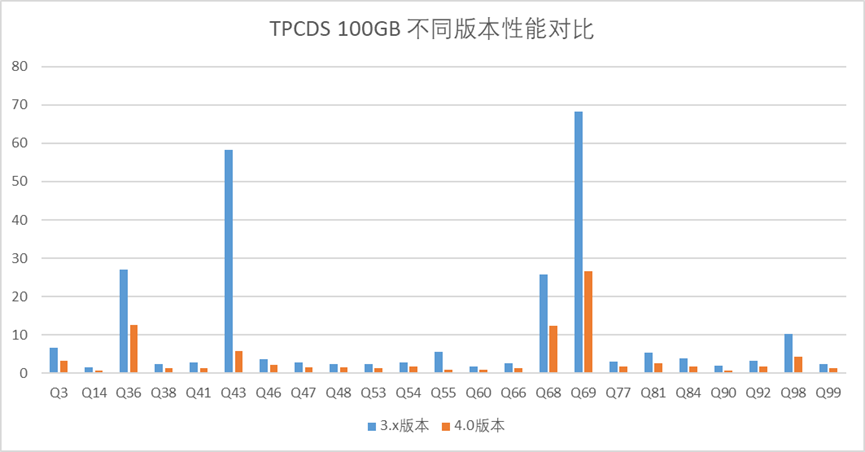TPC-DS 100GB 性能测试对比（OceanBase 3.x vs. 4.0)### OceanBase 数据库

github：https://github.com/oceanbase/oceanbase 欢迎大家

## 评论